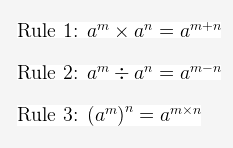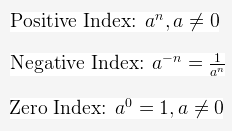# Theory of Logarithms: Laws of Logarithms and application of Logarithmic equations and indices

Welcome to class!

In today’s class, we will be talking about the theory of logarithms. Enjoy the class!

### Logarithm Theory#### Rules of logarithms

##### 1) Product Rule:

The logarithm of a product is the sum of the logarithms of the factors.

loga xy = loga x + loga y

##### 2) Quotient Rule:

The logarithm of a quotient is the logarithm of the numerator minus the logarithm of the denominator

loga (x/y) = loga x – loga y

loga xn nloga x

##### 4) Change of Base Rule:

loga b = logc b / logc a

loga b = 1 / logb a

where x and y are positive, and a > 0, a ≠ 1

###### Example:

Express log 2 4 + log 2 5 as a single logarithm

Solution

log 2 4 + log 2 5 = log 2 (4 × 5) = log 2 20

#### The properties of logarithms

1. loga 1 = 0 since a0 = 1.
2. loga a = 1 since a1 = a.
3. loga ax = x since ax = ax.

Product property: The log of a product equals the sum of the logs.
Quotient Property: The log of a quotient equals the difference of the logs.
Power Property: The log of power equals the product of the power and the log.

##### Logarithms applications
1. Population growth
2. Interest Rate Problems
3. Mortgage Problems
5. Earthquake Problems

#### Indices

##### Laws of Indices:Fractional indices:In our next class, we will be talking about Surds.  We hope you enjoyed the class.

Should you have any further question, feel free to ask in the comment section below and trust us to respond as soon as possible.

How Can We Make ClassNotesNG Better - CLICK to Tell Us💃

1.